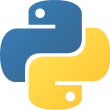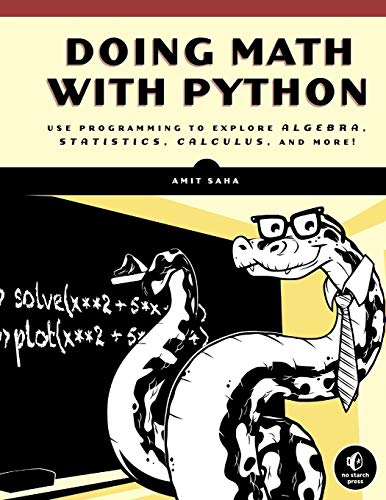Language
• Python 3Reading time
• Approximately 26 daysWhat you will learn
• Numerical Programming and Data MiningAuthor
• Amit SahaPublished
• 4 years, 11 months agoPackages you will be introduced to
• matplotlib
• sympy## Official description

Doing Math with Python shows you how to use Python to delve into high school–level math topics like statistics, geometry, probability, and calculus. You’ll start with simple projects, like a factoring program and a quadratic-equation solver, and then create more complex projects once you’ve gotten the hang of things.

Along the way, you’ll discover new ways to explore math and gain valuable programming skills that you’ll use throughout your study of math and computer science. Learn how to:
–Describe your data with statistics, and visualize it with line graphs, bar charts, and scatter plots
–Explore set theory and probability with programs for coin flips, dicing, and other games of chance
–Solve algebra problems using Python’s symbolic math functions
–Draw geometric shapes and explore fractals like the Barnsley fern, the Sierpinski triangle, and the Mandelbrot set
–Write programs to find derivatives and integrate functions

Creative coding challenges and applied examples help you see how you can put your new math and coding skills into practice. You’ll write an inequality solver, plot gravity’s effect on how far a bullet will travel, shuffle a deck of cards, estimate the area of a circle by throwing 100,000 "darts" at a board, explore the relationship between the Fibonacci sequence and the golden ratio, and more.

Whether you’re interested in math but have yet to dip into programming or you’re a teacher looking to bring programming into the classroom, you’ll find that Python makes programming easy and practical. Let Python handle the grunt work while you focus on the math.

Uses Python 3

## Write a review

Read this book? Comment on this book's GitHub issue page and share what you liked and what you didn't like about it. Your GitHub comment will show up as a review here. See an example.